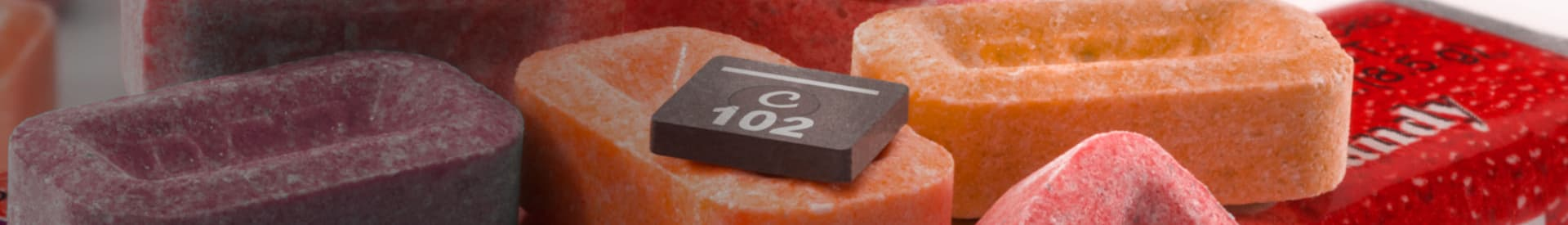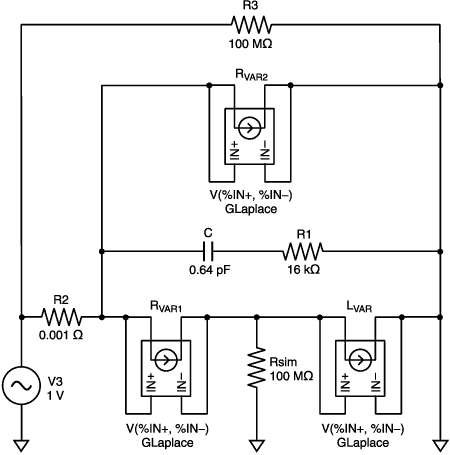## Site Settings

##### Currency settings
Products
RF
EMI
Resources
Product Documentation
Handling & Processing
Models & Layout Tools
Education
General
Video Library
Cx Family Common Mode Chokes
0402CT Low Profile Chip Inductors
XAL7050 High-inductance Shielded Power Inductors
XGL4020 Ultra-low DCR Power Inductors
Quality
Quality Certification
Material Certification
Safety Certification
Reliability
Handling / Processing
Careers
Locations
Resources# Using Coilcraft’s models in PSpice®

Note The models are based only on steady-state low-signal AC measurements and analysis. No DC bias or transient analyses were verified.

The GLaplace element is used in PSPICE to describe a frequency-dependent impedance. The impedance is given by the inverse of the XFORM, or XFORM = 1/Z(S).
1. Place a GLaplace element (part) into the model circuit schematic and connect as shown in the schematic below.
2. Edit the GLaplace element by double-clicking on the part in your schematic.
3. Click on the "XFORM =1/S" line and edit the value in the "value" box, as follows:

### For a frequency-dependent resistance (e.g. RVAR1, RVAR2)

Note: This example uses RVAR1 ( = k1 * sqrt(Frequency)). In your circuit, substitute the numerical value of k1 given in the model table for the specific model value you are using, into the XFORM statement.

`Z(S) = RVAR1 = k1*sqrt(Frequency), where Frequency is in degrees`

Since S is the frequency in radians, Frequency must be converted to degrees.

The resulting frequency-dependent resistance will be in Ohms units.

`1/Z(S) = XFORM = 1/k1/(−S*S/4/3.14159^2)^0.25`

### For a frequency-dependent inductance (e.g. LVAR)

Note: In your circuit, substitute the numerical values of k3, k4, and k5 given in the model table for the specific model value you are using, into the XFORM statement.

`Z = S*LVAR = k3-(k4*LOG(k5*Frequency)), where Frequency is in degrees`

The "LOG" function used here is the natural logarithm (base e, not base 10).

Since S is the frequency in radians, Frequency must be converted to degrees.

Since the inductance is given in uH units, the LVAR expression is converted as shown below.

`1/Z = XFORM = 1/(S*1e-6*( k3-(k4*LOG(k5*(S/(2*3.14159265))))))`

### Other circuit elements

Place and wire the other parts of the model in the schematic. If the specific model inductance element is a fixed value inductor, use the IND part instead of the GLaplace part. Edit the part values to match those of the model table values for the specific inductor you are simulating. See the example schematic and netlist shown below.

### Example PSpice schematic and netlist

In the case where two GLaplace elements are in series, a large-valued resistance to ground (Rsim) was added to prevent a floating node error. The large-valued resistance (R3) was added to measure voltage across the entire model.

Note: Make sure to substitute the specific model table values for each element (part) of the inductor model into the schematic.* Example Schematics Netlist *

```R_R2           \$N_0002 \$N_0001 0.001

V_V3           \$N_0002 0 DC 0V AC 1v

R_R3           \$N_0002 0 10meg

R_Rsim         0 \$N_0003 10meg

G_Rvar2        \$N_0001 0 LAPLACE { V(\$N_0001, 0) } {
+ 1/k2/(-S*S/4/3.14159^2)^0.25}

R_R1           \$N_0004 0 16000

G_Lvar         \$N_0003 0 LAPLACE { V(\$N_0003, 0) } {
+ 1/(S*1e-6*(k3-(k4*LOG(k5*(S/(2*3.14159265)))))) }

G_Rvar1        \$N_0001 \$N_0003 LAPLACE { V(\$N_0001, \$N_0003) } {
+ 1/k1/(-S*S/4/3.14159^2)^0.25}

C_C             \$N_0001 \$N_0004 .64pF```

### To view the specific effective simulation results

Include the following Macros in your Probe trace analysis to see frequency vs. inductance, impedance, phase angle (in degrees), and Q factor:

```PI = 3.14159265

L=(IMG(V(R3:1)/I(R2))/(2*pi*FREQUENCY)

Z = V(R3:1)/I(R2)

ANG = (180/PI)*ARCTAN((IMG(V(R3:1)/I(R2)))/(R(V(R3:1)/I(R2))))

QFACT = ABS((IMG(V(R3:1)/I(R2)))/(R(V(R3:1)/I(R2))))```